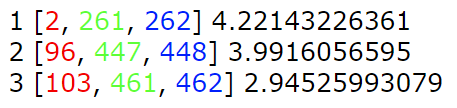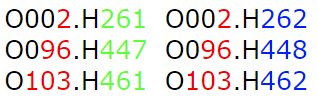# Difficult to extract data ****

Let's assume that we get such an output from a Python program. [2, 261, 262] is a list variable that is easy to print but kind off difficult to deal with in this form...

1 [2, 261, 262] 4.22143226361
2 [96, 447, 448] 3.9916056595
3 [103, 461, 462] 2.94525993079Use the data above and try to produce output like this (color images are for guidance)

O002.H261  O002.H262
O096.H447  O096.H448
O103.H461  O103.H462Posible solutions:

awk -F '[][,]' '{printf("O%03d.H%03d  O%03d.H%03d\n",$2,$3,$2,$4)}' data

or just by removing the problematic characters...
awk '{gsub(","," ",$0); gsub("\$"," ",0); gsub("\$"," ",$0); printf("O%03d.H%03d  O%03d.H%03d\n",$2,$3,$2,$4)}' data
# or with single gsub command
awk '{gsub(",\\|\\]\\|\\["," ",$0); printf("O%03d.H%03d O%03d.H%03d\n",$2,$3,$2,$4)}' data  Credits to Jonas Söderberg for the solution bellow: awk '{red = substr($2,2,length($2)-2); green = substr($3,1,length($3)-1); blue = substr($4,1,length(\$4)-1); printf( "O%03d.H%03d  O%03d.H%03d\n", red, green, red, blue)} data